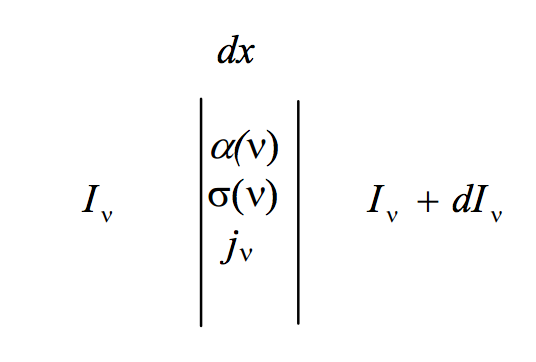# 5.5: The Equation of Transfer

$$\newcommand{\vecs}{\overset { \rightharpoonup} {\mathbf{#1}} }$$ $$\newcommand{\vecd}{\overset{-\!-\!\rightharpoonup}{\vphantom{a}\smash {#1}}}$$$$\newcommand{\id}{\mathrm{id}}$$ $$\newcommand{\Span}{\mathrm{span}}$$ $$\newcommand{\kernel}{\mathrm{null}\,}$$ $$\newcommand{\range}{\mathrm{range}\,}$$ $$\newcommand{\RealPart}{\mathrm{Re}}$$ $$\newcommand{\ImaginaryPart}{\mathrm{Im}}$$ $$\newcommand{\Argument}{\mathrm{Arg}}$$ $$\newcommand{\norm}{\| #1 \|}$$ $$\newcommand{\inner}{\langle #1, #2 \rangle}$$ $$\newcommand{\Span}{\mathrm{span}}$$ $$\newcommand{\id}{\mathrm{id}}$$ $$\newcommand{\Span}{\mathrm{span}}$$ $$\newcommand{\kernel}{\mathrm{null}\,}$$ $$\newcommand{\range}{\mathrm{range}\,}$$ $$\newcommand{\RealPart}{\mathrm{Re}}$$ $$\newcommand{\ImaginaryPart}{\mathrm{Im}}$$ $$\newcommand{\Argument}{\mathrm{Arg}}$$ $$\newcommand{\norm}{\| #1 \|}$$ $$\newcommand{\inner}{\langle #1, #2 \rangle}$$ $$\newcommand{\Span}{\mathrm{span}}$$$$\newcommand{\AA}{\unicode[.8,0]{x212B}}$$

The equation of transfer deals with the transfer of radiation through an atmosphere that is simultaneously absorbing, scattering and emitting.$$\text{FIGURE V.1}$$

Suppose that, between $$x$$ and $$x + dx$$ the absorption coefficient and the scattering coefficient at frequency $$\nu$$ are $$\alpha (\nu)$$ and $$\sigma (\nu)$$, and the emission coefficient per unit frequency interval is $$j_{\nu} d\nu$$. In this interval, suppose that the specific intensity per unit frequency interval increases from $$I_{\nu}$$ to $$I_{\nu} + dI_{\nu}$$ ($$dI_{\nu}$$ might be positive or negative). The specific intensity will be reduced by absorption and scattering and increased by emission. Thus:

$dI_{\nu} = - [I_{\nu} \alpha (\nu) + I_{\nu} \sigma (\nu) - j_{\nu} (\nu) ] dx. \label{5.5.1} \tag{5.5.1}$

This is one form - the most basic form - of the equation of transfer. Notice that $$\alpha$$ and $$\sigma$$ do not have a subscript.

This page titled 5.5: The Equation of Transfer is shared under a CC BY-NC 4.0 license and was authored, remixed, and/or curated by Jeremy Tatum via source content that was edited to the style and standards of the LibreTexts platform; a detailed edit history is available upon request.